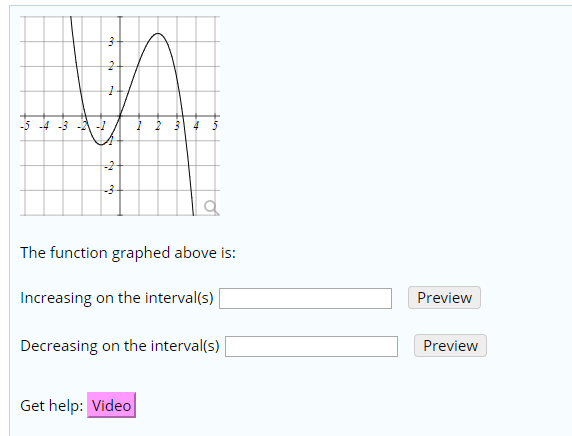# 2-5 -4 -3 - -4 5The function graphed above is:Increasing on the interval(s)PreviewDecreasing on the interval(s)PreviewGet help: Videoer

Question
1 viewshelp_outlineImage Transcriptionclose2 -5 -4 -3 - - 4 5 The function graphed above is: Increasing on the interval(s) Preview Decreasing on the interval(s) Preview Get help: Video er fullscreen
check_circle

Step 1

Given graph is:

From the graph, the critical points are at x = -1 and 2 as the graph changes here.

Now the domain is divided into three intervals based on the critical points i.e. (-∞,-1),(-1,2),(2,∞).

Consider the interval (-∞,-1):

As we move from left to right in the interval, the graph is moving downwards i.e. the value of the function is decreasing in this interval.

Con...

### Want to see the full answer?

See Solution

#### Want to see this answer and more?

Solutions are written by subject experts who are available 24/7. Questions are typically answered within 1 hour.*

See Solution
*Response times may vary by subject and question.
Tagged in

### Calculus